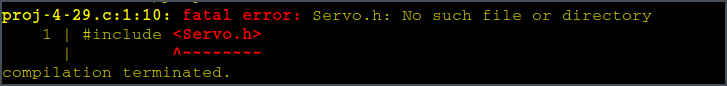# Arduino Servo.h no such file or directory on FreeBSD

So, here is the THING. I try to compile codes with Servo.h, but get No such file or directorys.Take a look at the compiling message, it looks like this.

...
-I/usr/local/arduino/libraries//usr/local/arduino/libraries
...

This is funny. It is generated by the vanilla arduino-mk file. So it has to be modified.

Check out CPPFLAGS.

CPPFLAGS
= -mmcu=$(MCU) -DF_CPU=$(F_CPU)
-DARDUINO=$(ARDUINO_VERSION) \ -I. -I$(ARDUINO_CORE_PATH)
-I$(ARDUINO_VAR_PATH)/$(VARIANT) \
$(SYS_INCLUDES)$(USER_INCLUDES) -g -Os -w -Wall \
-DUSB_VID=$(USB_VID) -DUSB_PID=$(USB_PID) \
-ffunction-sections -fdata-sections

Find the definition of SYS_INCLUDES and USER_INCLUDES

SYS_INCLUDES  = $(patsubst %,-I%,$(SYS_LIBS))
USER_INCLUDES = $(patsubst %,-I%,$(USER_LIBS))


Go on with SYS_LIBS and USER_LIBS.

SYS_LIBS  = $(patsubst %,$(ARDUINO_LIB_PATH)/%,$(ARDUINO_LIBS)) USER_LIBS =$(patsubst %,$(USER_LIB_PATH)/%,$(ARDUINO_LIBS))

Here, SYS_LIBS and USER_LIBS will replace ARDUINO_LIBS with ARDUINO_LIB_PATH/ARDUINO_LIBS and USER_LIB_PATH/ARDUINO_LIBS.

Go on with the definition of ARDUINO_LIB_PATH and USER_LIB_PATH.

ARDUINO_LIB_PATH  = $(ARDUINO_DIR)/libraries ifndef ARDUINO_SKETCHBOOK ARDUINO_SKETCHBOOK =$(HOME)/sketchbook
endif

ifndef USER_LIB_PATH
USER_LIB_PATH = $(ARDUINO_SKETCHBOOK)/libraries endif Problem lies here with ARDUINO_LIBS. According to the above definition ofSYS_INCLUDES and USER_INCLUDES. SYS_INCLUDES =$(patsubst %,-I%,$(SYS_LIBS)) USER_INCLUDES =$(patsubst %,-I%,$(USER_LIBS))  these will be replace in mk to be -I/usr/local/arduino/libraries//usr/local/arduino/libraries -I/home/username/sketchbook/libraries//usr/local/arduino/libraries All the lib files are located in /usr/local/arduino/libraries, so just refer to it directly will just be FINE. I don't want to specify the folder when doing the include thing (e.g. #include <Servo/Servo.h>)，so I added wildcard to include all the path under libraries folder. SYS_LIBS =$(wildcard $(patsubst %,%/**/*,$(ARDUINO_LIBS)))

And I need to add wildcard to the sources definition too. Otherwise the source code of included libraries won't be found.

LIB_C_SRCS    = $(wildcard$(patsubst %,%/**/*.c,$(SYS_LIBS))) LIB_CPP_SRCS =$(wildcard $(patsubst %,%/**/*.cpp,$(SYS_LIBS)))

It is safe to delete all the USER_LIBS part. Or just leave it be.

Next, I have to replace the libraries in/usr/local/arduino/libraries/ to the official newest arduino libs，the libs for BSD are too old, it just won't comile。Here is Arduino's github page.

Finally，I have to add some CPPFLAGS to correct the This library only supports blah blah blah error. This is due to the lack of ARDUINO_ARCH_AVR flag.

The final CPPFLAGS looks like this.

CPPFLAGS   =
-mmcu=$(MCU) -DF_CPU=$(F_CPU) -DARDUINO=$(ARDUINO_VERSION) \ -I. -I$(ARDUINO_CORE_PATH) -I$(ARDUINO_VAR_PATH)/$(VARIANT) \
$(SYS_INCLUDES)$(USER_INCLUDES) -g -Os -w -Wall -Wextra -std=gnu++11 \
-DUSB_VID=$(USB_VID) -DUSB_PID=$(USB_PID) \
-ffunction-sections -fdata-sections -fpermissive
-DARDUINO_AVR_UNO -DARDUINO_ARCH_AVR

I added the -std=gnu++11 and -DARDUINO_AVR_UNO -DARDUINO_ARCH_AVR part.

Voila！Enjoy arduino on FreeBSD.